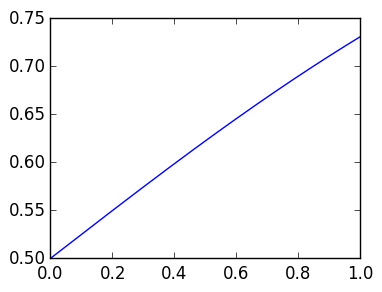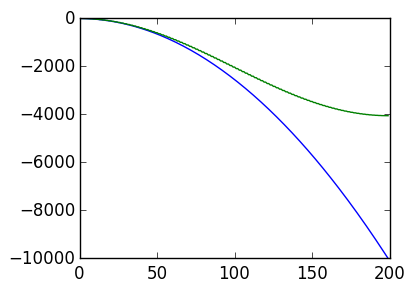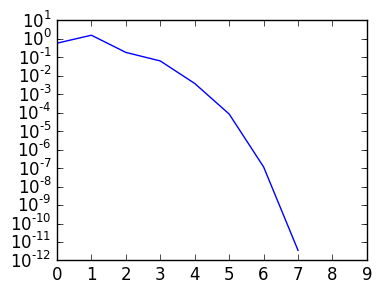# Lecture 39: CFL Condition, nonlinear boundary value problems¶

This lecture tidies up some loose ends. We will discuss very briefly Runge–Kutta methods, CFL Conditions, the secant method, Newton iteration in higher dimensions and nonlinear boundary value problems

## Runge–Kutta methods¶

We first mention that one should read up on Runge–Kutta methods, in particular RK4. This gives an explicit (like forward Euler) time-stepping scheme that converges like $O(h^4)$. That is, if we approximate

$$u'=f(t,u)$$

by $w_k$ where $w_{k+1}$ is found using RK4, with a step-size of $h$, then if we evolve to a time $T = nh$ where $h=T/n$, we have

$$w_n - u(T) = O(h^4).$$

This is a substantial improvement over forward Euler, which only achieved $O(h)$ convergence.

We can define RK4 as follows:

In :
function RK4(L,y,h)
k1=L(y)
k2=L(y+.5h*k1)
k3=L(y+.5h*k2)
k4=L(y+h*k3)

y+h*(k1+2k2+2k3+k4)/6.
end

Out:
RK4 (generic function with 1 method)

Here we solve the logistic equation $u' = u(1-u)$:

In :
w=zeros(1001)
w=0.5
h=0.001
for k=1:length(w)-1
w[k+1]=RK4(u->u*(1-u),w[k],h)
end

using PyPlot
plot((0:h:1.),w);## The CFL Condition¶

Here we explain roughly why we need to take $\Delta t \leq C \Delta x^2$ for some constant $C$ when using explicit methods for time stepping. Consider the periodic Heat equation

$$u_t = u_{\theta\theta}$$

If we solve it with forward Euler and finite differences in space, we get

$$\mathbf w_{k+1} = \mathbf w_k + (\Delta t) \tilde D_n^2 \mathbf w_k$$

Consider the eigenvalue decomposition

$$\tilde D_n^2 = Q \Lambda Q^\top$$

Then define

$$\mathbf z_k \triangleq Q^\top \mathbf w_k$$

so that

$$\mathbf z_{k+1} = \mathbf z_k + (\Delta t) \Lambda \mathbf z_k$$

Each entry is thus evolved independentally:

$$\mathbf e_j^\top \mathbf z_{k+1} = \mathbf e_j^\top \mathbf z_k + (\Delta t) \lambda_j \mathbf e_j^\top \mathbf z_k$$

The eigenvalues grow roughly like $\lambda_j \sim -\tilde C j^2$:

In :
function DL(n)
h=2π/n
ret=zeros(n,n)
for k=1:n-1
ret[k,k]=-1
ret[k,k+1]=1
end
ret[n,1]=1
ret[n,n]=-1
ret/h
end

function DR(n)
h=2π/n
ret=zeros(n,n)
for k=2:n
ret[k,k]=1
ret[k,k-1]=-1
end
ret[1,n]=-1
ret[1,1]=1
ret/h
end
n=200

Δθ=(2π)/n

D2=DL(n)*DR(n)

plot(-(1:n).^2/4)
plot(reverse(eigvals(D2)));For forward Euler to be stable evolving

$$u' = a u$$

,

we observed that we needed a step size satisfying $h \leq { C_1 \over |a|}$ for some constant $C_1$.

In the previous case, its equivalent to evolving $u' = \lambda_j u$, hence we need

$$\Delta t \leq { C_1 \over \max |\lambda_j|} \leq {C_2 \over n^2} = C_3 \Delta x^2.$$

## Secant method¶

An issue with the Newton step

$$x_{k+1} = x_k - {f(x_k) \over f'(x_k)}$$

is that it requires knowing the derivative of $f$. We can get around this by approximating

$$f'(x_k) \approx {f(x_k) - f(x_{k-1}) \over x_k - x_{k-1}}$$

$$x_{k+1} = x_k - {f(x_k) (x_k-x_{k-1}) \over f(x_k)-f(x_{k-1})}$$

Note we need to specify both $x_0$ and $x_1$. We see that it still converges quite quickly:

In :
f=x->x^2-2.

x=zeros(10)
x=2.
x=3.
for k=2:9
x[k+1]=x[k]-f(x[k])*(x[k]-x[k-1])/(f(x[k])-f(x[k-1]))
end

semilogy(x-sqrt(2.))Out:
1-element Array{Any,1}:
PyObject <matplotlib.lines.Line2D object at 0x3144fab10>

## Higher-dimensional Newton iteration¶

Suppose we have $f : \mathbb R^n \rightarrow \mathbb R^n$ and we want to find $\mathbf r$ satisfying $f(\mathbf r) = 0$. We can do so using Newton iteration, where the derivative is replaced by the Jacobian.

Let's consider the $n=2$ case, where we want to solve

$$f_1(x,y)=0, f_2(x,y)=0$$

The Jacobian is

$$J(x) = \begin{pmatrix} {\partial f_1 \over \partial x} & {\partial f_2 \over \partial x} \cr {\partial f_1 \over \partial y} & {\partial f_2 \over \partial y}\end{pmatrix}$$

Then Newton iteration is

$$\mathbf x_{k+1} = \mathbf x_k - J(\mathbf x_k)^{-1} f(\mathbf x_k)$$

We can try it out:

In :
f=(x,y)->[x^2-y^2-2; x*y+3]
J=(x,y)->[2x -2y; y x]

x0=[1.,2.]
x=x0

for k=1:10
x=x-J(x...)\f(x...)
end

x,f(x...)

Out:
([-2.040166086417569,1.470468517231287],[-8.881784197001252e-16,0.0])

## Newton iteration for boundary value problems¶

Consider now a nonlinear boundary value problem:

$$u'' +xu +u^2 = 0, u(0) = a, u(1)=b$$

We can think of this as a root-finding problem $N(u) = 0$ where

$$N(u) = \begin{pmatrix} u(0)-a \cr D^2 u + xu +u^2 \cr u(1)-b\end{pmatrix}$$

Formally, we can differentiate this with respect to u to get an operator:

$$N'(u) = \begin{pmatrix} B_0 \cr D^2 + x +2u \cr B_1\end{pmatrix}$$

where $B_x u\triangleq u(x)$. We obtain a Newton iteration

$$u_{k+1} = u_k - N'(u_k)^{-1}N(u_k)$$

where $N'(u_k)^{-1}$ is interpreted as solving a linear boundary value problem.

We can implement this in practice:

In :
using ApproxFun

u0=Fun(0.,[0.,1.])
D=Derivative(space(u0))
x=Fun(space(u0))

u=u0

a=1.;b=0.

for k=1:10
u = u-  [dirichlet();D^2+x+2u]\[u(0.)-a;u(1.)-b; u''+x*u+u^2]
end

ApproxFun.plot(u)

Out:
In :
norm( u''+x*u+u^2)

Out:
1.2389381059027248e-16

Exercise Re-implement this using finite section.